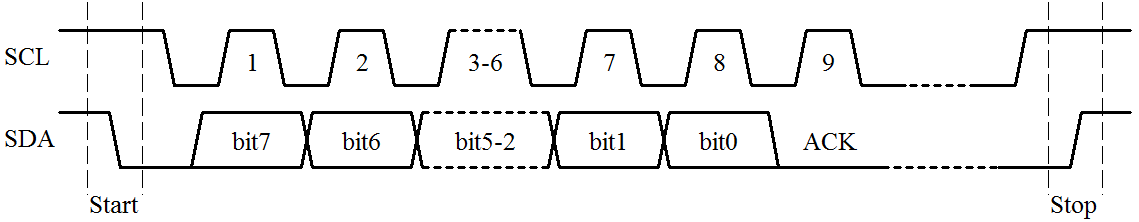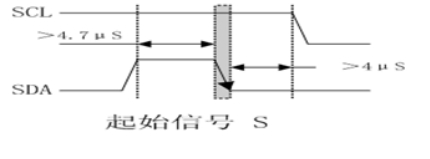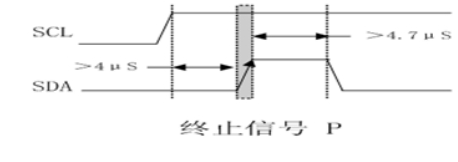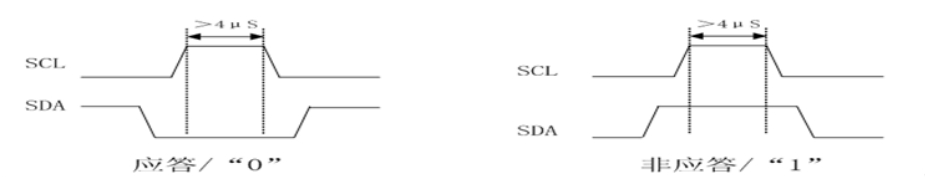### IIC简介

IIC 是一种通信协议，是一种串行通信结构，由一根时钟线 SCL 以及一根数据线 SDA 组成，在 I2C 总线传输过程中，将两种特定的情况定义为开始和停止条件：当SCL 保持“高”时，SDA 由“高”变为“低”为开始条件；当 SCL 保持“高”且 SDA 由“低”变为“高”时为停止条件。void IIC_GPIO_Init() {
//定义GPIO结构体
GPIO_InitTypeDef GPIO_InitStruct;
//打开GPIOB时钟
RCC_APB2PeriphClockCmd(RCC_APB2Periph_GPIOB,ENABLE);
//设置时钟引脚和数据引脚
GPIO_InitStruct.GPIO_Pin = SCL_PIN | SDA_PIN;
//设置为推挽输出
GPIO_InitStruct.GPIO_Mode = GPIO_Mode_Out_PP;
//设置输出速率为50MHz
GPIO_InitStruct.GPIO_Speed = GPIO_Speed_50MHz;
//初始化
GPIO_Init(GPIO_PORT,&GPIO_InitStruct);
}

void IIC_SDA_Mode(u8 mode) {
GPIO_InitTypeDef GPIO_InitStruct;
if (mode) {//1、写数据
GPIO_InitStruct.GPIO_Pin = SDA_PIN;
GPIO_InitStruct.GPIO_Mode = GPIO_Mode_Out_PP;
GPIO_InitStruct.GPIO_Speed = GPIO_Speed_50MHz;
GPIO_Init(GPIO_PORT,&GPIO_InitStruct);
} else {//0、代表读数据
GPIO_InitStruct.GPIO_Pin = SDA_PIN;
GPIO_InitStruct.GPIO_Mode = GPIO_Mode_IPU;
GPIO_Init(GPIO_PORT,&GPIO_InitStruct);
}
}

SCL在高电平期间，SDA由高电平向低电平的变化定义为起始信号IIC起始信号代码实现

void IIC_start() {
//设置为输出模式，输出
IIC_SDA_Mode(OUT);
//将时钟线SCK和数据线SDA拉高
IIC_SDA_1;
IIC_SCK_1;
delay_us(5);
//SCK处于高电平的状态下SDA由高变低产生开始信号
IIC_SDA_0;
delay_us(5);
//将SCK拉低准备传输数据
IIC_SCK_0;
}

SCL在高电平期间，SDA由低电平向高电平的变化定义为停止信号IIC停止信号代码实现

void IIC_stop() {
//设置为输出模式，输出
IIC_SDA_Mode(OUT);
IIC_SDA_0;
delay_us(5);
IIC_SCK_1;
delay_us(5);
IIC_SDA_1;
}IIC应答信号代码实现

u8 IIC_Wait_Ack(void) {
u8 times = 0;
IIC_SDA_Mode(INPUT);//设置为输入模式，主机要读取从机的应答信号
IIC_SCK_1;
delay_us(5);
//等待应答，如果250次后没有应答则发送结束信号
if (++times > 250) IIC_stop();
return 1;
}
IIC_SCK_0;
delay_us(5);
return 0;
}

IIC写一字节数据

void IIC_write(u8 date) {
u8 i, temp;
temp = date;
IIC_SCK_0;//将时钟线拉低，传输数据
delay_us(5);
for(i = 0; i < 8; i++) {
IIC_SDA_Mode(OUT);
if (((temp << i) & 0x80) == 0 ) {//ox80 = 1000 0000
IIC_SDA_0;//表示数据位是0
} else {
IIC_SDA_1;//表示数据位是1
}
IIC_SCK_1;//将SCK拉高
delay_us(5);
IIC_SCK_0;//将SCK拉低
delay_us(5);
}
}

IIC读一字节数据

u8 IIC_read(void) {
u8 i, temp = 0;
for(i = 0; i < 8; i++) {
IIC_SDA_Mode(INPUT);
IIC_SCK_1;//将时钟线拉高，只有SCL为高时数据有效
delay_us(5);
temp <<= 1;
}